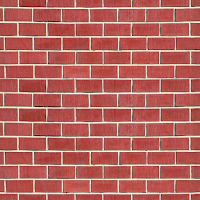## Videos

» » » How to calculate number of Bricks, Cement and Sand for Brickwork Construction ?

# How to calculate number of Bricks, Cement and Sand for Brickwork Construction ?Brickwork Construction
In most of the building construction projects brick is used in an higher proportion nearly about 70 to 80%. So, estimating the quantity of bricks and other material for construction is the basic step to do construction in an economy way.

1. Bricks used in the construction is available in different sizes based on the location for our present study let us take a standard size of  modular brick is 190 X 90 X 90 mm.
2. Assume Mortar thickness as 10 mm
3. Cement mortar ratio 1:6
4. Calculation is done for Brickwork volume 1cum (1 cubic metre) - Volume of your brickwork
Calculation

Volume of bricks with mortar

Volume of 1 brick with mortar = 200 X 100 X 100 ( 10 mm mortar thickness on all sides)

= 0.2 X 0.1 X 0.1

Volume of brick with mortar = 0.002 Cum (m3)

Number of Bricks required for 1 cubic metre = 1/0.002 = 500 Numbers

Volume of bricks without mortar

Volume of 1 brick without mortar = 190 X 90 X 90

= 0.19 X 0.09 X 0.09 (converting to metre)

Volume of 1 brick without mortar  =  0.001539 Cum (m3)

Volume of 500 bricks without mortar = 500 X 0.001539 Cum

Volume of bricks without mortar for 1 cum = 0.7695 Cum  (m3)

Required amount of cement mortar = 1 Cum – Volume of bricks without mortar

= 1 – 0.7695

Required amount of Cement Mortar  = 0.2305 Cum  (m3) (Wet Condition)

The Calculated volume is in a wet condition that means we need 0.2305 cum cement mortar in mixed condition (after mixing water). In order to find the dry volume, we need to multiply 33 % as bulkage of sand.

Dry volume of a mortar = 0.2305 cum X 1.33 = 0.306565 cum

Mortar Ratio is 1:6
1 part cement and 6 part sand, total part 1+6 =7
Density of cement = 1440 kg, multiplying the 1440 kg density of cement with dry volume of mortar to calculate the cement quantity.
1= cement part
7= total
Required amount Cement quantity in brickwork = 0.306565 X 1/7 X 1440 kg

Required amount Cement quantity = 63 Kg = 1.26 bags
Approximately 1.26 bags if the bacg is 50 Kg

6 = Sand part
7 = total
Required amount of Sand = 0.306565 X 6/7 = 0.26277 Cubic metre

For 1Cubic metre of Brickwork, we require

• 500 Bricks
• 63 Kg Cement
• 0.263 cum sand

Android app for Brickwork Calculator
Windows Version